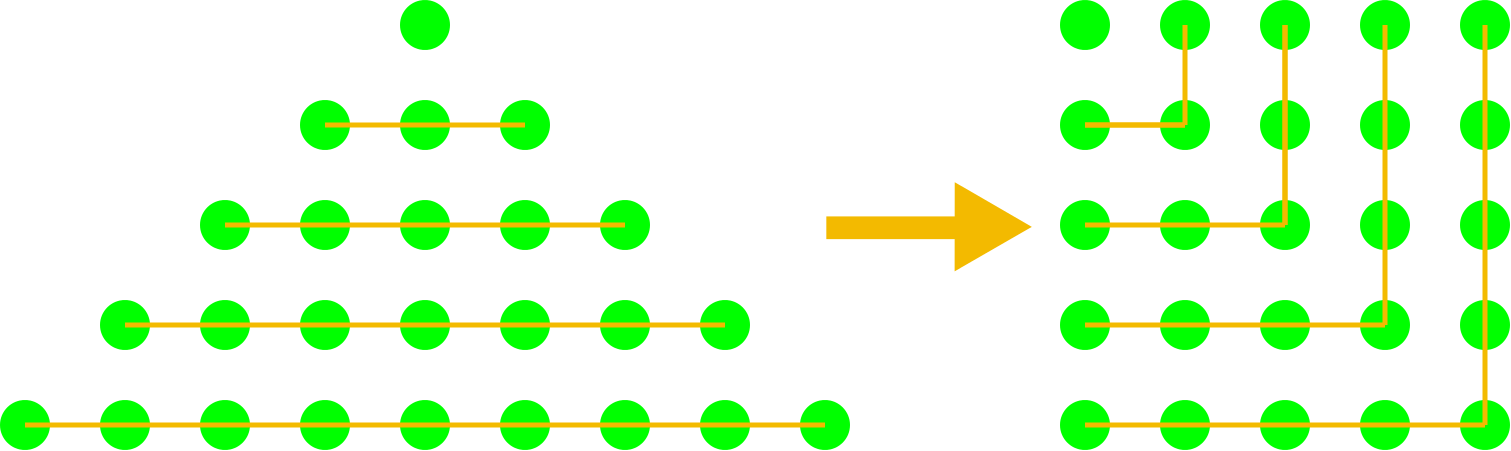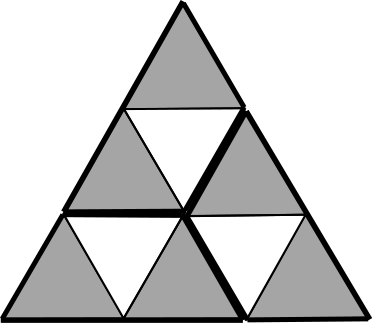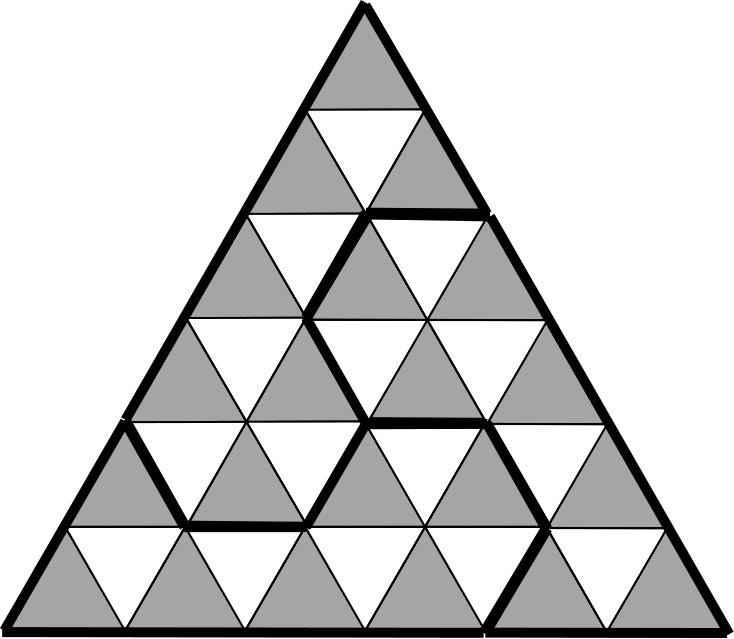#### You may also like### Dividing the Field

A farmer has a field which is the shape of a trapezium as illustrated below. To increase his profits he wishes to grow two different crops. To do this he would like to divide the field into two trapeziums each of equal area. How could he do this?### Two Circles

Draw two circles, each of radius 1 unit, so that each circle goes through the centre of the other one. What is the area of the overlap?### Star Gazing

Find the ratio of the outer shaded area to the inner area for a six pointed star and an eight pointed star.

# Trisected Triangle

##### Age 14 to 16 Short Challenge Level:

If an equilateral triangle is split into a number of smaller identical equilateral triangles then there must be one small triangle on the top row, three small triangles on the row below, five small triangles in the row below that and so on.

So total number of small triangles is $1$ or $4$ or $9$ or $16$ etc. These are exactly the square numbers, the diagram below might help you to understand why.So, for three copies of a tile to form an equilateral triangle, the number of triangles which comprise the tile must be one third of a square number.

Of the four given tiles, only the third and fourth satisfy this condition, and both of these tiles can make equilateral triangles:This problem is taken from the UKMT Mathematical Challenges.
You can find more short problems, arranged by curriculum topic, in our short problems collection.